### Defining the Terms

Mesh Size: Refers to a mesh scale of a particle size of a granular material, also referred to as grain size.

Particle: An individual grain of clay.

Platelet: The thickness of a clay particle, which can also define its physical shape.

Specific Surface Area (SSA): A property of solids as the total surface area of a particle per unit of mass.

SSA Values: Based on platelet size and organized in ascending values: 17 is the largest platelet and 28 is the smallest.

### Specific Surface Area

For decades, stoneware clay formulation has centered on particle size distribution (PSD). To achieve density, this concept progressively arranged particle sizes from the largest to the smallest, in the tightest order possible. Optimizing the packing density (measuring the particles that fit into a certain volume) was thought to produce the tightest body, which would result in the highest vitrification values. Even well-planned and calculated formulas still produced bodies that had weeping issues and never achieved the desired level of porosity. While PSD is still the primary formulation criteria, it’s better to base it on Specific Surface Area (SSA).

Ultimately, clay particles are not one size or shape, and particle size is only one of the parameters important to creating water-tight clay bodies. Industry measures both particle size and platelet size to give the more accurate measure of SSA (also called SAS). In simpler terms, SSA is the total surface area of a material per unit of mass (square meters per gram, for example). It combines the total of the face (particle size) and the depth (platelet size) into a singular value. Although potters might know the particle sizes they are blending, the platelet depth may be unknown to them because of the lack of data from the supplier(s).

To further illustrate, in common potters language, a 200-mesh particle of clay might have a depth of either 1600 mesh or 200 mesh. The SSA value takes this particle size variance into account by assigning ascending values based on platelet size. A smaller number indicates a larger platelet, and a higher number indicates a smaller platelet.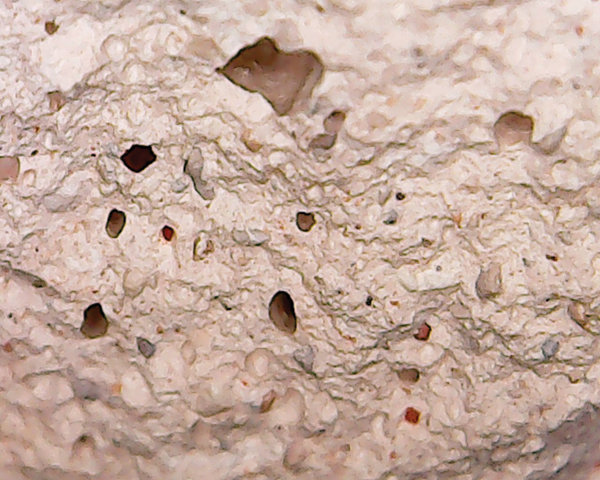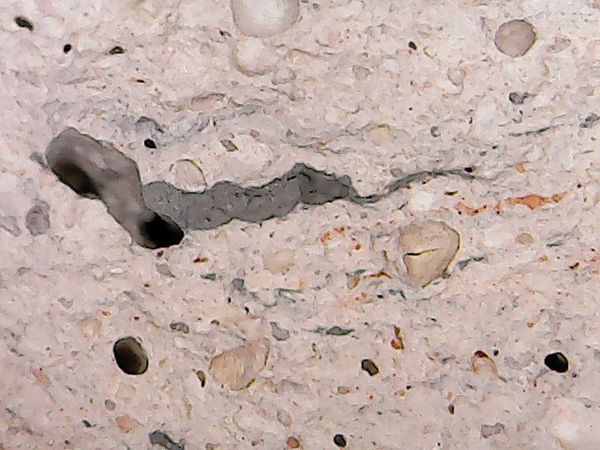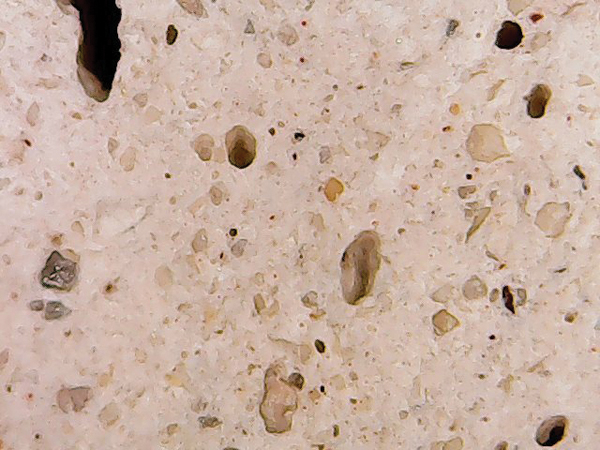The SSA numerical value is, in essence, telling the potter how thick the platelet is on any given clay particle. A thick platelet is a lower number and a thin platelet is a higher number (25). Bringing these numbers into a visual reference, then, means 17 would be a cube, whereas 28 would be paper thin. Any given bag of clay is going to have a range of sizes, but the average particle size is given as median PSD. So while you have selected 200-mesh clay, the SSA will tell you if that mesh size is cube-like, or paper thin. Unfortunately, SSA values can only be found on MSDS sheets, or on the manufacturers’ websites.

Selecting clay by mesh size does not necessarily mean you have selected a smaller overall clay particle. A 325-mesh clay with an SSA of 18 is roughly the same size as a 200-mesh clay with an SSA of 24. This is of particular importance when formulating stoneware clay bodies because they begin with large particle sizes that range from 20–80 mesh. The higher the percentages of large particles involved in a formula, the more critical it becomes to have higher SSA valued clays to fill in the voids. If 200- and 325-mesh clays are added, and both have an SSA value of 17, you have filled in the round blanks with cubes.

Therefore, the historic industry standard of PSD is applicable to stoneware formulation. On the other hand though, using PSD as the sole basis would be inaccurate because PSD does not account for platelet size. To achieve density packing, 200–400-mesh clays can be added, but if all those additions have low SSA values, voids are still present. As cone firing temperatures decrease, and high percentages of large particle fireclays increase, the percentage of high-valued SSA particles must increase.

### Formulation

Stoneware and porcelain formulation have never been calculated with formula limits, not the way one sees with glazes. Stoneware has been more the product of potters experimenting with various combinations of fireclay, intermediate-, and fine-mesh clays to achieve a workable body. Most stoneware clay bodies, at least those that I have studied, fall into the 80/10/10 category: 80% clays, 10% silica, and 10% feldspar. Although there is a wide variation in mesh formulation, the totality of the formula remains unchanged.

Fine tuning the formula (after testing the initial recipe), usually includes adding enough intermediate (and fine) meshes to lower absorption, which will make the fired clay body water tight. If formulation is for non-functional use, then PSD is far less critical. For functional stoneware, the ultimate goal is to achieve vitrification. To achieve this, PSD becomes the primary formulation criteria, and SSA value becomes the basis of that formula.

### Converting Recipes

Table 1 (shown below) demonstrates how to convert common stoneware formulas from PSD to SSA average. You’ll need to know the parts/percentage of the material, as well as the SSA value for each. To help with the second step, after the recipe is converted, the materials are also placed into four categories or groups: large-mesh clay, intermediate-mesh clay, silica, and feldspar.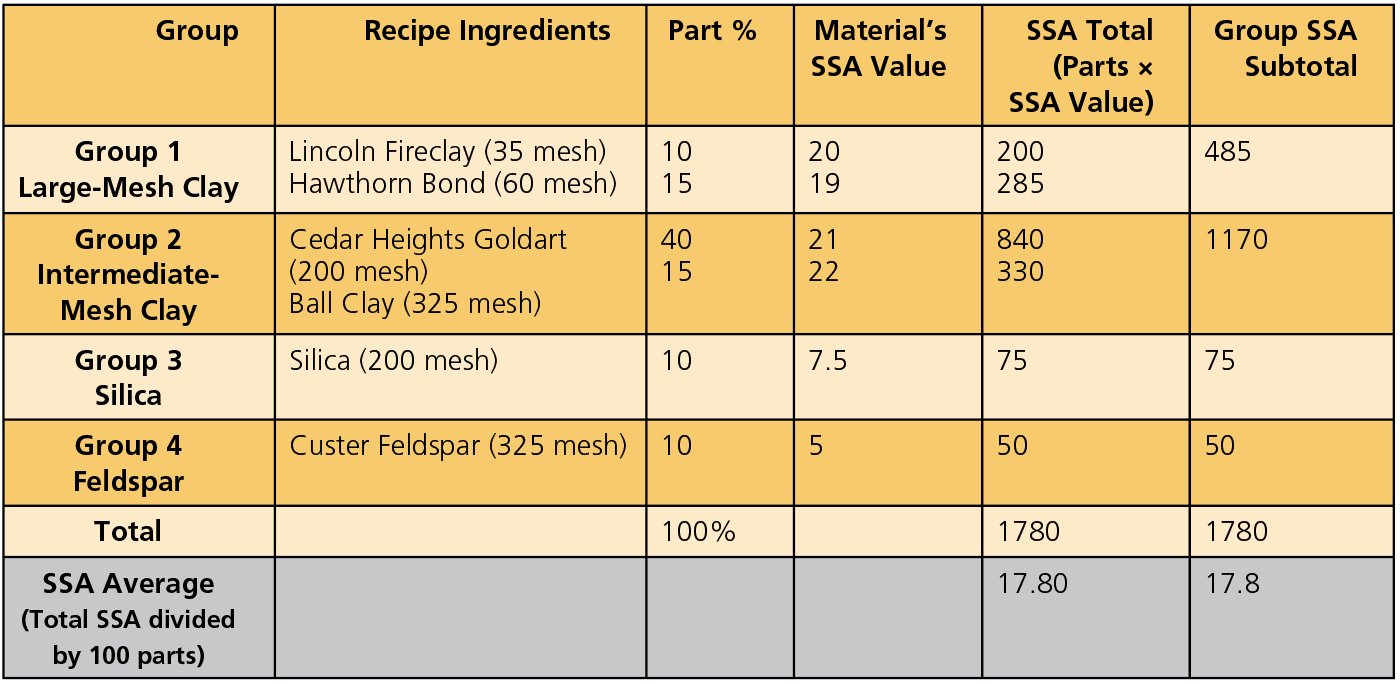In using the PSD method, the potter needs to calculate and reconcile the percentages of each mesh size in order to achieve maximum PSD. Assigning SSA values to each addition results in a total SSA value. The potter then simply divides the total SSA by the 100-part equation to determine a median SSA. Now, instead of estimating the percentages of mesh sizes, the potter has a hard SSA value, in this case, of 17.80. The next step is to determine if the median SSA value of 17.80 is appropriate for this particular formula. Once that is determined, adjustments can be made.

### Determining Appropriate SSA

• Analyze the four groups of materials, listed in table 1. The SSA formula requires that silica (group 3) and feldspar (group 4) remain constant, because silica and feldspar are set by other formula limit parameters. (It requires a specific amount of feldspar to achieve an effective melt, and it requires a specific amount of silica to maintain the appropriate silica/alumina ratio. So the SSAs for those categories are deducted from the total SSA (1780 minus 125 equals 1655 SSA). This new SSA value represents clay content only.
• The heart of any stoneware formula is fireclay, and formulas can vary widely in their percentage of use. As shown in table 1, large-particle fireclay makes up 25 parts (a quarter) of the total recipe. Since this provides the clay body’s dominant characteristics, the combined SSA values (485) for the two fireclays used in this sample recipe are deducted from the total.
• After deducting silica (group 3), and feldspar (group 4), we have 1655 SSA. After deducting 485 SSA (group 1, large-mesh clay), that leaves 1170 SSA for group 2.
• Group 2 is comprised of 40 parts Cedar Heights Goldart and 15 parts ball clay, giving a combined total of 55 parts.
• The final computation for the group 2 SSA formula requires a simple calculation of dividing the remaining 1170 SSA by 55 parts (see table 2): 1170 divided by 55 (parts) equals 21.72 median SSA value. SSA values typically run from 17 (coarse) up to 28, which is ultra fine.

Based on this information and absorption tests on this cone 10 clay body, the SSA value of 21.72 would be appropriate for non-functional use. For functional use, an SSA value of 23.50 should be achieved by changing your percentages of Cedar Heights Goldart and ball clay (for optimal density and an absorption rate of 2%).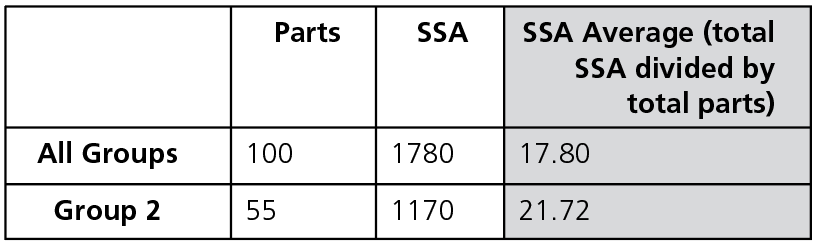### Steps for Evaluating Appropriate SSA

1. Divide materials into four groups, identify quantities of materials in each group, and calculate the SSA total for all four.

2. Identify constants (silica and feldspar) and subtract them from the SSA total (cone 10 recipe shown in table 1).

3. Next, identify materials used in group 1, providing the prominent characteristics associated with stoneware.

4. As shown in table 2 (group 2), the remaining SSA value is achieved by dividing the SSA (1170) by the parts (55). An SSA average of 23.50 is desirable for functional use.

the author Thomas Anderson has spent the last decade researching technical research papers from various universities on clay formulation and is now writing formula limits for clay chemistry.

Topics: Pottery Clay